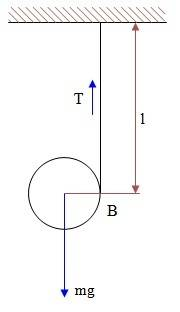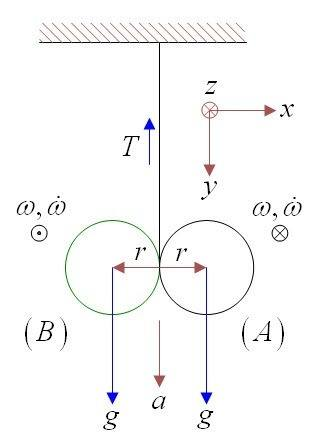# Yo-yo pulled to stay in place

## Homework Statement[/B] A yo-yo of mass m and radius r starts to roll from rest till it reaches the end of it's thread B.
1) What are the COM and angular velocities and accelerations at B.
2) What is the tension Td in the wire during the descend?
3) What is the tension Tu in the wire during the ascend?
4) On a time, a boy pulls the string. at what angular acceleration will the yo-yo rotate if the COM stays in place?
5) At which pace must the boy pull the string in order to keep the COM in place.
6) If the string's length is l, how long can this situation last?

## Homework Equations

Kinetic energy of a solid body: ##E_k=\frac{1}{2}I\omega^2##
Shteiner's theorem: ##I_c=I_{c.o.m.}+Mr^2##
Moment of inertia of a ball round it's center: ##I_{cen}=\frac{2}{5}mr^2##
Torque and angular acceleration: ##M=I\alpha##
In accordance with motion with constant acceleration: ##\omega^2=2\dot\omega\theta##

3. The Attempt at a Solution

During descent and at B:
$$r\cdot mg=(I_c+mr^2)\dot\omega~~\rightarrow~~\dot\omega=\frac{mgr}{I_c+MR^2}$$
The center's acceleration: ##\dot y=\dot\omega r=\frac{mgr^2}{I_c+MR^2}##
The total angle m has traveled along the string's length l is ##\theta=\frac{l}{2\pi r}2\pi=\frac{l}{r}##
The angular velocity at B:
$$\omega_B^2=2\dot\omega_B\theta=\frac{2mlg}{I_c+mr^2}$$
The linear velocity: ##v_B=\omega_B\cdot r##
The tension Td during descent:
$$mg-T_d=m\dot y=mr\dot\omega~~\rightarrow~~T_d=mg\left( 1-\frac{mr^2}{I_c+mr^2} \right)$$
The tension Tu during ascend is the same since the force-acceleration equation ##mg-T_d=m\dot y## is the same.
4) In order to keep the center in place the boy must pull with a force F that equals: ##F=mg## , so the torque round the center is:
$$F\cdot r=mg\cdot r=I_c\dot\omega~~\rightarrow~~\dot\omega=\frac{mgr}{I_c}$$
5) The boy must pull the string with acceleration of ##\dot y=\dot\omega r##
6) The string's length is l and from kinematics:
$$l=\frac{1}{2}\dot\ y t^2=\frac{1}{2}\frac{mgr^2}{I_c}t^2~~\rightarrow~~t^2=\frac{2lI_c}{mgr^2}$$

haruspex
Homework Helper
Gold Member
You quote the MoI for a ball, but yoyos are normally more cylindrical, in my experience.

You can simplify the expression for Td.

You've used ##\dot y## for linear acceleration. You mean ##\ddot y##, I guess. In the final line, the dot seems to have slipped off the y.

Otherwise, all looks good.Descending:
$$m\vec g-\vec T=m\vec a=m(\vec\alpha\times\vec r)$$
$$\rightarrow~~mg-T=m\dot\omega r~~\rightarrow~~T=mg\left[ 1-\frac{mr^2}{I_A} \right]$$
In ascending, m rotates to the other side of the string, the green disk.
The same vector formula, because ##\dot{\vec\omega}## points to the negative side of the z axis yields:
$$mg-T=-m\dot\omega r~~\rightarrow~~T=mg\left[ 1+\frac{mr^2}{I_A} \right]$$
If it's true i don't understand. the velocity doesn't appear, so only the acceleration counts. it could have been that situation (B) is the first, i mean descending and then T would be the same, greater? it seems to me that only the side of the string has changed.

haruspex
Homework Helper
Gold Member
View attachment 102396 Descending:
$$m\vec g-\vec T=m\vec a=m(\vec\alpha\times\vec r)$$
$$\rightarrow~~mg-T=m\dot\omega r~~\rightarrow~~T=mg\left[ 1-\frac{mr^2}{I_A} \right]$$
In ascending, m rotates to the other side of the string, the green disk.
The same vector formula, because ##\dot{\vec\omega}## points to the negative side of the z axis yields:
$$mg-T=-m\dot\omega r~~\rightarrow~~T=mg\left[ 1+\frac{mr^2}{I_A} \right]$$
If it's true i don't understand. the velocity doesn't appear, so only the acceleration counts. it could have been that situation (B) is the first, i mean descending and then T would be the same, greater? it seems to me that only the side of the string has changed.
My mistake, you were right the first time. The tension will be the same.
In the analysis above, both ##\vec \alpha## and ##\vec r ## change sign.

Thank you haruspex